# Connecting Strain Gages to a DAQ Device

## Before You Begin

This document provides step-by-step instructions for wiring and configuring your DAQ device for strain gage measurements. Before you begin using your NI DAQ hardware, you must install your application development environment and NI-DAQmx driver software. Refer to the Installing LabVIEW and NI-DAQmx document for more information.

### Strain Measurement Fundamentals

You can measure strain with a strain gage, which is a device with electrical resistance that varies in proportion to the amount of strain in the device, and with signal conditioning. When using a strain gage, you bond the strain gage to the device under test, apply force, and measure the strain by detecting changes in resistance (Ω). Strain gages return varying voltages in response to stress or vibrations in materials. Resistance changes in parts of the strain gage to indicate deformation of the material. Strain gages require excitation, generally voltage excitation, and linearization of the voltage measurements.

Strain measurements rarely involve quantities larger than a few microstrain (µε). Therefore, measuring strain requires accurate measurements of very small changes in resistance. For example, if a test specimen undergoes a substantial strain of 500 µε, a strain gage with a gage factor of 2 exhibits a change in electrical resistance of only 2 × (500 × 10 -6 ) = 0.1%. For 120 Ω, this is a change of only 0.12 Ω.

### Wheatstone Bridges

To measure such small changes in resistance and compensate for temperature sensitivity, strain gages often use a Wheatstone bridge configuration with a voltage or current excitation source. The general Wheatstone bridge, shown in the following figure, is a network of four resistive legs with an excitation voltage, VEX, that is applied across the bridge. One or more of these legs can be active sensing elements.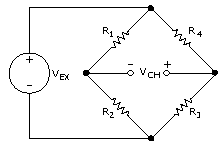Figure 1. Wheatstone Bridge

The Wheatstone bridge is the electrical equivalent of two parallel voltage divider circuits. R1 and R2 compose one voltage divider circuit, and R3 and R4 compose the second voltage divider circuit. You measure the output of a Wheatstone bridge between the middle nodes of the two voltage dividers.

A physical phenomenon, such as a temperature shift or a change in strain applied to a specimen, changes the resistance of the sensing elements in the Wheatstone bridge. You can use the Wheatstone bridge configuration to help measure the small variations in resistance that the sensing elements produce corresponding to a physical change in the specimen.

### Gage Factor

A fundamental parameter of the strain gage is its sensitivity to strain, expressed quantitatively as the gage factor. Gage factor is the ratio of the fractional change in electrical resistance to the fractional change in length, or strain. The gage factor must be the same for each gage in the bridge.

The gage factor for metallic strain gages is usually around 2. You can obtain the actual gage factor of a particular strain gage from the sensor vendor or sensor documentation.

### Nominal Gage Resistance

Nominal gage resistance is the resistance of a strain gage in an unstrained position. You can obtain the nominal gage resistance of a particular gage from the sensor vendor or sensor documentation. The resistance across each arm of the bridge must be the same for the bridge to be unstrained. For example, if you have two strain gages and two reference resistors, the gages must have the same nominal gage resistance, and the resistance of the reference resistors must be the same as the nominal gage resistance for the strain gages.

### Types of Strain Gages

Strain-gage configurations are arranged as Wheatstone bridges. The gage is the collection of all of the active elements of the Wheatstone bridge. There are three types of strain-gage configurations: quarter-bridge, half-bridge, and full-bridge. The number of active element legs in the Wheatstone bridge determines the kind of bridge configuration. You can measure axial strain, bending strain, or both.

 Configuration Number of Active Elements Quarter-bridge 1 Half-bridge 2 Full-bridge 4

Table 1. Strain Gage Configurations

NI-DAQmx strain virtual channels use the following equation to scale voltage readings to strain units.

Vr = (VCH / VEX)STRAINED – (VCH / VEX)UNSTRAINED

where VEX is the excitation voltage, and VCH is the measured voltage.

#### Quarter-Bridge Type I

The following figure shows how to position a strain gage resistor in axial and bending configurations for the quarter-bridge type I.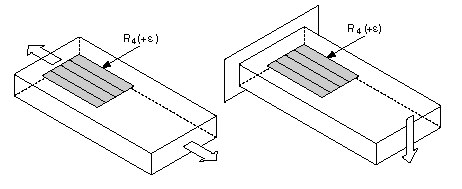Figure 2. Quarter-Bridge Type 1 Configuration

Quarter-bridge type I strain gage configurations have the following characteristics:

• A single active strain gage element mounted in the principal direction of axial or bending strain.
• A passive quarter-bridge completion resistor, known as a dummy resistor, in addition to half-bridge completion.
• Temperature variation decreasing the accuracy of the measurements.
• Sensitivity at 1000 µε is ~ 0.5 mVout / VEX input.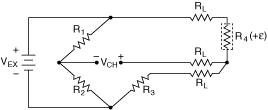Figure 3. Quarter-Bridge Type I Circuit Diagram

The following symbols apply to the circuit diagram in Figure 3:

• R1 is the half-bridge completion resistor.
• R2 is the half-bridge completion resistor.
• R3 is the quarter-bridge completion resistor, known as a dummy resistor.
• R4 is the active strain gage element measuring tensile strain (+ε).
• VEX is the excitation voltage.
• RL is the lead resistance.
• VCH is the measured voltage.

#### Quarter-Bridge Type II

The following figure shows how to position a strain gage resistor in axial and bending configurations for the quarter-bridge type II.Figure 4. Quarter-Bridge Type II Configuration

Quarter-bridge type II strain gage configurations have the following characteristics:

• One active strain gage element and one passive, temperature-sensing quarter-bridge element, known as a dummy resistor. The active element is mounted in the direction of axial or bending strain. The dummy gage is mounted in close thermal contact with the strain specimen but is not bonded to the specimen, and is usually mounted transverse, or perpendicular, to the principle axis of strain. This configuration is often confused with the half-bridge type I configuration, but in the half-bridge type I configuration, the R3 element is active and bonded to the strain specimen to measure the effect of Poisson's ratio.
• Completion resistors which provide half-bridge completion.
• Compensation for temperature.
• Sensitivity at 1000 µε is ~ 0.5 mVout / VEX input.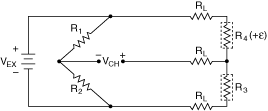Figure 5. Quarter-Bridge Type II Circuit Diagram

The following symbols apply to the circuit diagram:

• R1 is the half-bridge completion resistor.
• R2 is the half-bridge completion resistor.
• R3 is the quarter-bridge temperature sensing element, known as a dummy resistor.
• R4 is the active strain gage element measuring tensile strain (+ε).
• VEX is the excitation voltage.
• RL is the lead resistance.
• VCH is the measured voltage.

#### Half-Bridge Type I

The following figure shows how to position strain gage resistors in axial and bending configurations for the half-bridge type I.Figure 6. Half-Bridge Type I Configuration

Half-bridge type I strain gage configurations have the following characteristics:

• Two active strain gage elements, one mounted in the direction of axial strain and the other acting as a Poisson gage and mounted transverse, or perpendicular, to the principal axis of strain.
• Completion resistors that provide half-bridge completion.
• Sensitivity to both axial and bending strain.
• Compensation for temperature.
• Compensation for the aggregate effect on the principal strain measurement due to the Poisson's ratio of the material.
• Sensitivity at 1000 µε is ~ 0.65 mVout/ VEX input.Figure 7. Half-Bridge Type I Circuit Diagram

The following symbols apply to the circuit diagram:

• R1 is the half-bridge completion resistor.
• R2 is the half-bridge completion resistor.
• R3 is the active strain gage element measuring compression due to the Poisson effect (–ε).
• R4 is the active strain gage element measuring tensile strain (+ε).
• VEX is the excitation voltage.
• RL is the lead resistance.
• VCH is the measured voltage.

#### Half-Bridge Type II

The half-bridge type II configuration measures only bending strain. The following figure shows how to position strain gage resistors in a bending configuration for the half-bridge type II.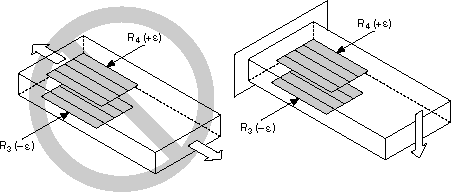Figure 8. Half-Bridge Type II Configuration

Half-bridge type II strain gage configurations have the following characteristics:

• Two active strain gage elements, one mounted in the direction of axial strain on the top side of the strain specimen and the other mounted in the direction of axial strain on the bottom side.
• Completion resistors that provide half-bridge completion.
• Sensitivity to bending strain.
• Rejection of axial strain.
• Compensation for temperature.
• Sensitivity at 1000 µε is ~ 1 mVout / VEX input.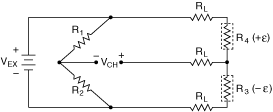Figure 9. Half-Bridge Type II Circuit Diagram

The following symbols apply to the circuit diagram:

• R1 is the half-bridge completion resistor.
• R2 is the half-bridge completion resistor.
• R3 is the active strain gage element measuring compressive strain (–ε).
• R4 is the active strain gage resistor measuring tensile strain (+ε).
• VEX is the excitation voltage.
• RL is the lead resistance.
• VCH is the measured voltage.

#### Full-Bridge Type I

The full-bridge type I configuration measures only the bending strain. The following figure shows how to position strain gage resistors in a bending configuration for the full-bridge type I.Figure 10. Full-Bridge Type I Configuration

Full-bridge type I strain gage configurations have the following characteristics:

• Four active strain gage elements, two mounted in the direction of bending strain on the top side of the strain specimen and the other two mounted in the direction of bending strain on the bottom side.
• High sensitivity to bending strain.
• Rejection of axial strain.
• Compensation for temperature.
• Sensitivity at 1000 µε is ~ 2.0 mVout / VEX input.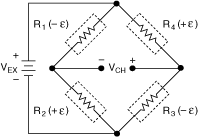Figure 11. Full-Bridge Type I Circuit Diagram

The following symbols apply to the circuit diagram:

• R1 is the active strain gage element measuring compressive strain (–ε).
• R2 is the active strain gage element measuring tensile strain (+ε).
• R3 is the active strain gage element measuring compressive strain (–ε).
• R4 is the active strain gage element measuring tensile strain (+ε).
• VEX is the excitation voltage.
• RL is the lead resistance.
• VCH is the measured voltage.

#### Full-Bridge Type II

The full-bridge type II configuration measures only bending strain. The following figure shows how to position strain gage elements in a bending configuration for the full-bridge type II.Figure 12. Full-Bridge Type II Configuration

Full-bridge type II strain gage configurations have the following characteristics:

• Four active strain gage elements. Two are mounted in the direction of bending strain with one on the top side of the strain specimen and the other on the bottom side. The other two act together as a Poisson gage and are mounted transverse, or perpendicular, to the principal axis of strain with one on the top side of the strain specimen and the other on the bottom side.
• Rejection of axial strain.
• Compensation for temperature.
• Compensation for the aggregate effect on the principal strain measurement due to the Poisson's ratio of the material.
• Sensitivity at 1000 µε is ~ 1.3 mVout / VEX input.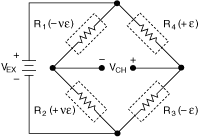Figure 13. Full-Bridge Type II Circuit Diagram

The following symbols apply to the circuit diagram:

• R1 is the active strain gage element measuring compressive Poisson effect (–ε).
• R2 is the active strain gage element measuring tensile Poisson effect (+ε).
• R3 is the active strain gage element measuring compressive strain (–ε).
• R4 is the active strain gage element measuring tensile strain (+ε).
• VEX is the excitation voltage.
• RL is the lead resistance.
• VCH is the measured voltage.

#### Full-Bridge Type III

The following figure shows how to position strain gage resistors in an axial configuration for the full-bridge type III. The full-bridge type III configuration measures only the axial configuration.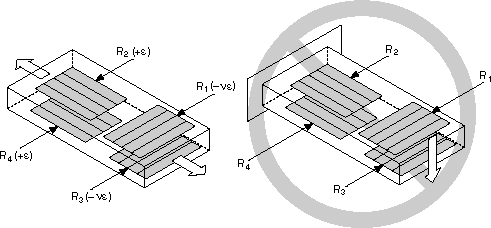Figure 14. Full-Bridge Type III Configuration

Full-bridge type III strain gage configurations have the following characteristics:

• Four active strain gage elements. Two are mounted in the direction of axial strain with one on the top side of the strain specimen and the other on the bottom side. The other two act together as a Poisson gage and are mounted transverse, or perpendicular, to the principal axis of strain with one on the top side of the strain specimen and the other on the bottom side.
• Compensation for temperature.
• Rejection of bending strain.
• Compensation for the aggregate effect on the principle strain measurement due to the Poisson's ratio of the material.
• Sensitivity at 1000 µε is ~ 1.3 mVout / VEX input.Figure 15. Full-Bridge Type III Circuit Diagram

The following symbols apply to the circuit diagram:

• R1 is the active strain gage element measuring compressive Poisson effect (–ε).
• R2 is the active strain gage element measuring tensile strain (+ε).
• R3 is the active strain gage element measuring compressive Poisson effect (–ε).
• R4 is the active strain gage element measuring tensile strain (+ε).
• VEX is the excitation voltage.
• RL is the lead resistance.
• VCH is the measured voltage.

### Signal Conditioning Requirements for Strain Gages

Common signal conditioning requirements for strain gages are bridge completion, bridge excitation, excitation sensing, signal amplification, offset nulling, shunt calibration, and linearization. You should calibrate your strain gage periodically to account for changes in the physical characteristics of the strain gage and in the material the gage is mounted to, to account for variations in the leadwire resistance, and to compensate for imperfections in the measurement system. Calibrating strain gages usually involves two steps: offset nulling, or bridge balancing, and shunt calibration, or gain adjustment.

#### Bridge Completion

Unless you are using a full-bridge strain gage sensor with four active gages, you must complete the bridge with reference resistors. Therefore, strain gage DAQ devices typically provide half-bridge completion networks consisting of two high-precision reference resistors. The nominal resistance of the completion resistors is less important than how well the two resistors match. Ideally, the resistors match well and provide a stable reference voltage of VEX/2 to the negative input lead of the measurement channel. The high resistance of the completion resistors helps minimize the current draw from the excitation voltage.

#### Bridge Excitation

Strain gage DAQ devices typically provide a constant voltage source to power the bridge. Though there is no standard voltage level that is recognized industry wide, excitation voltage levels of around 3 V and 10 V are common.

#### Excitation Sensing

If the strain gage circuit is located away from the DAQ device and excitation source, a possible source of error is voltage drops caused by resistance in the wires that connect the excitation voltage to the bridge. Therefore, some DAQ devices include a feature called remote sensing to compensate for this error. There are two common methods of remote sensing. With feedback remote sensing, you connect extra sense wires to the point where the excitation voltage wires connect to the bridge circuit. The extra sense wires regulate the excitation supply, compensate for lead losses, and deliver the needed voltage at the bridge. An alternative remote sensing scheme uses a separate measurement channel to measure directly the excitation voltage delivered across the bridge. Because the measurement channel leads carry very little current, the lead resistance has a negligible effect on the measurement. You then can use the measured excitation voltage in the voltage-to-strain conversion to compensate for lead losses.

#### Signal Amplification

The output of strain gages and bridges is relatively small. In practice, most strain gage bridges and strain-based transducers output less than 10 mV/V, or 10 millivolts of output per volt of excitation voltage. Therefore, strain gage DAQ devices usually include amplifiers to boost the signal level, increase measurement resolution, and improve signal-to-noise ratios.

#### Offset Nulling (Bridge Balancing)

When you install a strain gage, the gage probably will not output exactly 0 V when no strain is applied. Slight variations in resistance among the bridge legs generate some nonzero initial offset voltage. A system can handle this initial offset voltage in a few different ways.

##### Software Compensation

This method of bridge balancing compensates for the initial voltage in software. With this method, you take an initial measurement before the strain input is applied. You then can use this initial voltage in the strain equations. This simple and fast method requires no manual adjustments. The disadvantage of the software compensation method is that the method does not remove the offset of the bridge. If the offset is large enough, it limits the amplifier gain you can apply to the output voltage, thus limiting the dynamic range of the measurement.

##### Offset Nulling Circuit

The second bridge balancing method uses an adjustable resistor, or potentiometer, to electrically adjust the output of the bridge to 0 V.

##### Hardware Nulling Compensation

The third method, like the software compensation method, does not affect the bridge directly. A nulling circuit adds an adjustable DC voltage, positive or negative, to the output of the instrumentation amplifier to compensate for initial bridge offset. Refer to the device documentation to determine the hardware nulling methods your DAQ device provides.

You can verify the output of a strain gage measurement system by comparing the measured strain with a calculated strain value if the physical strain on the strain gage is known. The difference (if any) between the calculated and the measured strain can then be used for each measurement as a gain adjustment factor. If not all parameters of a strain measurement are known, you can simulate a mechanical strain by connecting a large known resistor in parallel with the strain gage. This resistor, called a shunt resistor, offsets the zero voltage of the bridge. Because the value of the shunt resistor is known, you can calculate the mechanical strain corresponding to the voltage drop of the resistor. You can then compare this voltage to the voltage output of the strain gage undergoing the same mechanical strain. This gain adjustment factor (calibration factor) can then be applied to every measurement.

## Locating Your DAQ Device Pinout

Before connecting any signals, locate your device pinout.

1. Open Measurement & Automation Explorer (MAX) and expand Devices and Interfaces.
2. Right-click on your device name, and select Device Pinouts.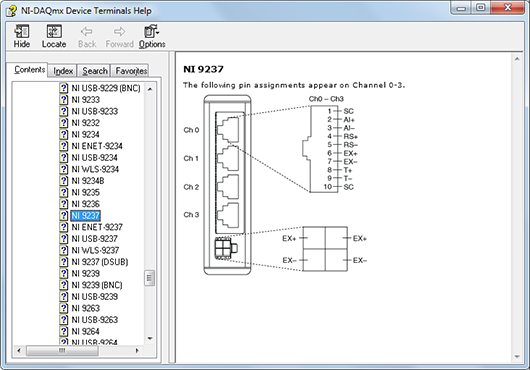Figure 16. Device Terminals Help

## Configuring a Strain Measurement

You can use MAX to quickly verify the accuracy of your measurement system setup. Using an NI-DAQmx Global Virtual Channel, you can configure a strain measurement without any programming. A virtual channel is a concept of the NI-DAQmx driver architecture used to represent a collection of device property settings that can include a name, a physical channel, input terminal connections, the type of measurement or generation, and scaling information.

1. With MAX open, select Data Neighborhood and click Create New.
2. Select NI-DAQmx Global Virtual Channel and click Next.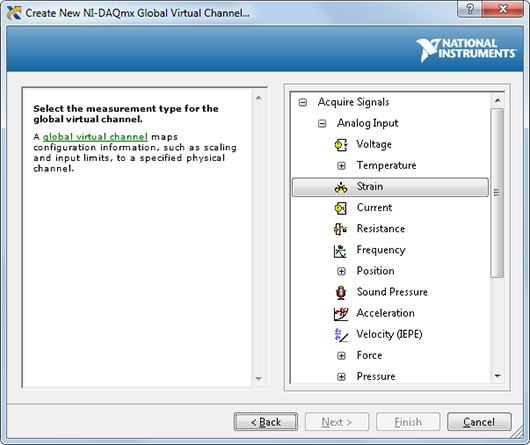Figure 17. Creating an NI-DAQmx Virtual Channel

1. Select ai0 or whichever physical channel you intend to use to connect your strain gage. A physical channel is a terminal or pin at which you can measure or generate an analog or digital signal. A single physical channel can include more than one terminal or pin, as in the case of a differential input channel.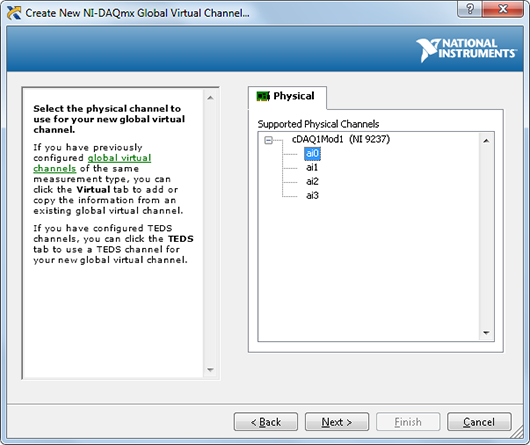Figure 18. Device Physical Channels

1. Click Next and enter a name for the global virtual channel or leave the default.
2. Click Finish and you should see the following screen in MAX: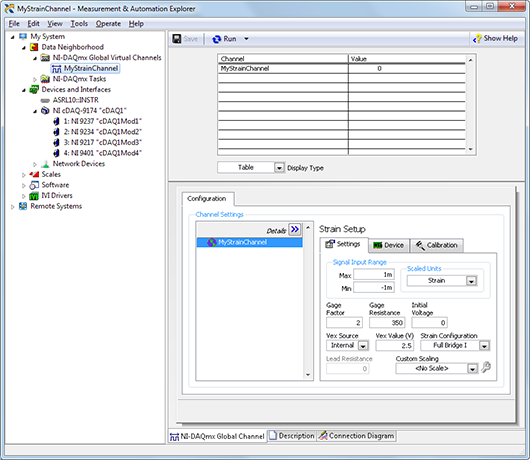Figure 19. Setting Up a Strain Channel in MAX

1. On the Settings tab, type in the minimum and maximum strain values you expect to read from your strain gage (-1m to 1m by default).
2. Configure the Gage Factor, Gage Resistance, Initial Voltage, VEX Source, VEX Value (V), Lead Resistance, and Strain Configuration based on your sensor specifications and the information discussed above in the Strain Measurement Fundamentals section.

## Wiring a Strain Gage to Your Device

The next step is to physically connect the strain gage to your DAQ device.

1. Click the Connection Diagram tab in MAX to continue.Figure 20. Strain Connection Diagram

The connection diagram above indicates which pins on your DAQ device should be wired according to the physical channel you selected. In this example, a full bridge type I configuration uses pins 2, 3, 6, and 7, corresponding to AI+, AI-, EX+, and EX- on an NI 9237 C Series module.

## Strain Gage Calibration

When you configure a strain measurement task, you can use the Strain Gage Calibration Wizard to calibrate your strain gage. Complete the following steps to calibrate the strain gage:Figure 21. Strain Gage Calibration Wizard

1. Select the Device tab and click Calibrate to launch the Strain Gage Calibration Wizard.
2. Follow the steps on the Set Up Hardware window. You can configure the following settings:
1. Enable Offset Nulling — Select Enable Offset Nulling to perform an offset nulling calibration procedure.
2. Enable Shunt Calibration — Select Enable Shunt Calibration to perform a shunt calibration procedure. If you select this option, configure the following settings:
1. Shunt Resistor Value — Specify the exact resistor value of the shunt resistor. The default value is the resistance of the shunt resistor that is the factory shipping default for your hardware. For the greatest accuracy, use a 6½ digit digital multimeter to measure the resistance of your entire shunt calibration circuit with the shunt switch engaged and leads connected. This increases the accuracy of your measurements by compensating for the shunt enable switch resistance and any lead resistance caused by long lead wires. However, since the shunt resistor value is very large, these do not greatly impact the measurement accuracy.
2. Shunt Resistor Location — Specify the location on the Wheatstone bridge to which the shunt resistor is connected. Refer to the figure on the Set Up Hardware window for the relative positions of R1, R2, R3 and R4.
3. Click Next.Figure 22. Measure and Calibration Window

1. Use the Measure and Calibrate window to measure and calibrate your strain gage. The software automatically takes the first measurement, and the table displays the results. The table shows the following information:
1. Channel Information
1. Channel Name—The name of the virtual channel on which strain calibration is performed.
2. Physical Chan—The physical channel to which the strain gage is connected.
2. Offset Adjustment—This section shows information about the offset error to help you determine if you should perform an offset null calibration. Perform an offset null calibration if you perform a shunt calibration.
1. Meas Val (strain)—The measured offset value with units of strain. Normally, this value should be close to zero.
2. Err (%)—Percentage offset error, determined by the equation: Err (%) = [(offset value * 100) / (Max Range LimitMin Range Limit)] where Max Range Limit and Min Range Limit are the maximum and minimum strain range values specified for the given virtual channel.
3. Gain Adjustment—This section shows values related to gain error and the shunt calibration procedure:
1. Sim Val (strain)—The simulated strain value based on the hardware Set Up and the shunt resistor value.
2. Meas Val (strain)—The measured strain value. Normally, this value should be close to the simulated strain value.
3. Gain Adj Val—The gain adjust value. This value is the end result of the shunt calibration and is used to scale strain measurements on this channel. Normally, this value is close to 1, which implies that the measured value and simulated value are equal. Your strain channel stores the gain adjust value as the AI.Bridge.ShuntCal.GainAdjust attribute.
4. Err (%)—Percentage gain error, determined by the equation: Err (%) = (measured value – simulated value) * 100 / (MaxMin) where Max and Min are the maximum and minimum strain values specified for the given virtual channel.
4. Perform your measurement and calibration with the following buttons:
1. Click Measure to make a preliminary measurement using the offset and shunt calibration data from a previously run strain gage procedure.
2. Click Reset Data to reset the offset and shunt calibration data and make a measurement using their default values.
3. Click Calibrate to perform the offset and/or shunt calibration. The table shows the resulting measurements.
5. Click Finish when you are done. The calibration data is saved as part of your virtual channel. On successful completion, the software automatically uses the calibration data when scaling voltage to strains on this virtual channel.

## Testing the Signal

Use NI-DAQmx global virtual channels to preview your measurements.

1. With MAX still open, click back on the NI-DAQmx Global Channel tab and click on the Run button to see the strain value of your measurement displayed at the top of the screen.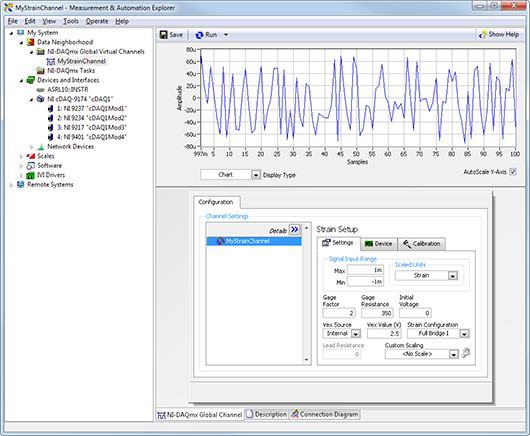Figure 23. Previewing a Strain Measurement in MAX

You can choose to view the signal in tabular form or as a graph by selecting Graph from the Display Type dropdown. You also have the option of saving your NI-DAQmx Global Virtual Channel should you wish to refer to this configuration screen again in the future.

Prev Connect and Set Up Hardware
Introduction to LabVIEW Next